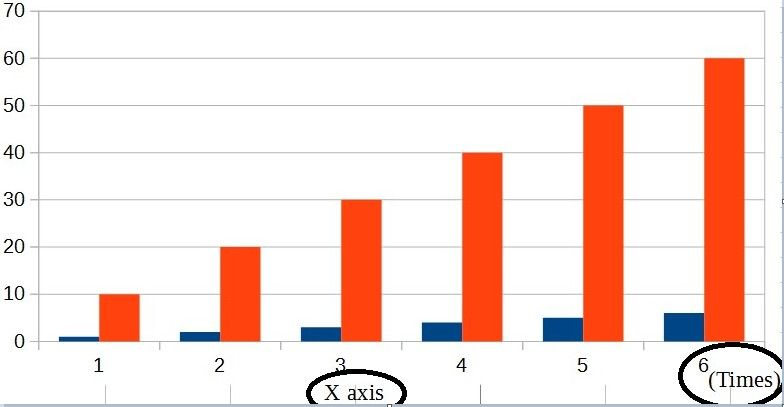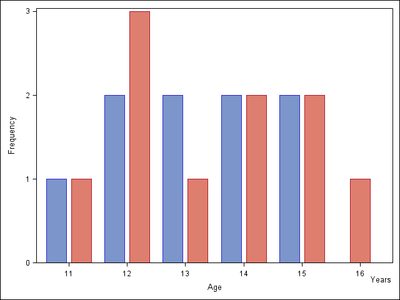## can sas plot this graph?

Hi all,

I would like to plot graph as  below.

X-axis has 2 labels.

what's the command i should do?

proc sgplot data=tmp;
series x=rownum y=avg ;

xaxis label="X axis";

run;1 ACCEPTED SOLUTION

Accepted Solutions

## Re: can sas plot this graph?

You probably want to combine the LABEL= option for the XAXIS statement with teh global FOOTNOTE statement.

Experiment with using the FOOTNOTE JUSTIFY= option to get what you want.

You might also need to move the legend around. Here's an example to get started:

``````footnote justify=center "X axis";
proc sgplot data=sashelp.class;
vbar age / group=sex response=height stat=mean groupdisplay=cluster;
xaxis label="(Time)" labelpos=right;
keylegend / position=topleft location=inside;
run;``````

3 REPLIES 3

## Re: can sas plot this graph?

What version of SAS do you have?

Have you looked at annotate?

## Re: can sas plot this graph?

You probably want to combine the LABEL= option for the XAXIS statement with teh global FOOTNOTE statement.

Experiment with using the FOOTNOTE JUSTIFY= option to get what you want.

You might also need to move the legend around. Here's an example to get started:

``````footnote justify=center "X axis";
proc sgplot data=sashelp.class;
vbar age / group=sex response=height stat=mean groupdisplay=cluster;
xaxis label="(Time)" labelpos=right;
keylegend / position=topleft location=inside;
run;``````PGStats
Opal | Level 21

## Re: can sas plot this graph?

Here is how to do this with SG annotation:

``````data anno;
function="text";
drawspace="layoutpercent";
anchor="topright";
x1=100; y1=7;
label="Years";
run;

proc sgplot data=sashelp.class noautolegend sganno=anno;
vbar age / group=sex groupdisplay=cluster barwidth=0.8;
run;``````PG
Discussion stats
• 3 replies
• 1158 views
• 6 likes
• 4 in conversation